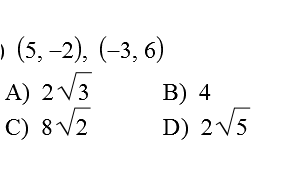GeoB blast 4
Period *
simplify *
1 point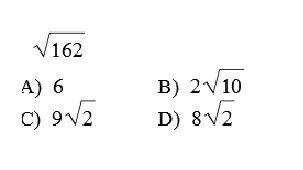Find x *
1 point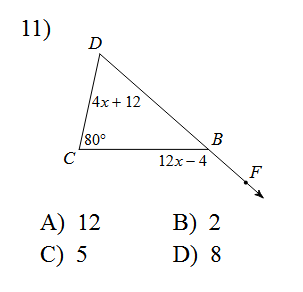Find the distance between the two points *
1 point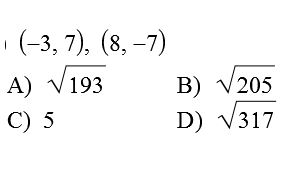find x *
1 point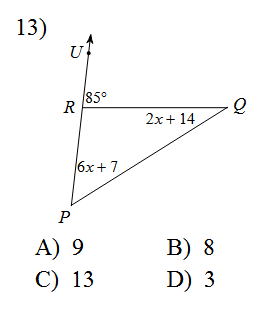find x *
1 point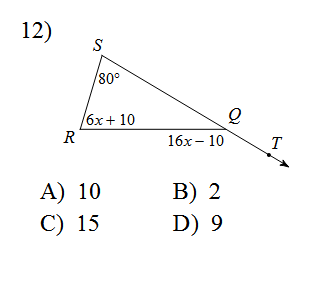Find the distance *
1 point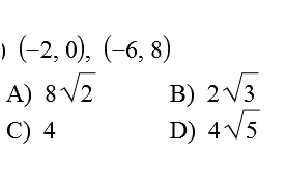simplify *
1 point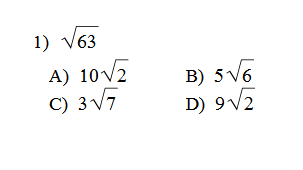Find the angle *
1 pointFind the distance between these two points *
1 point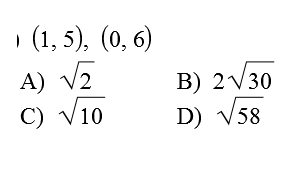simplify *
1 point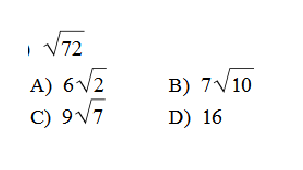simplify *
1 point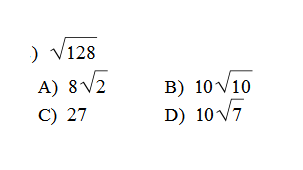Find the angle *
1 point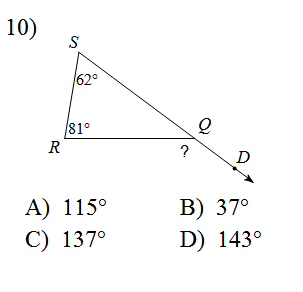Last Name *